# 系统编程-进程-close-on-exec机制

PART1

exec系列函数功能简介exec系列函数登场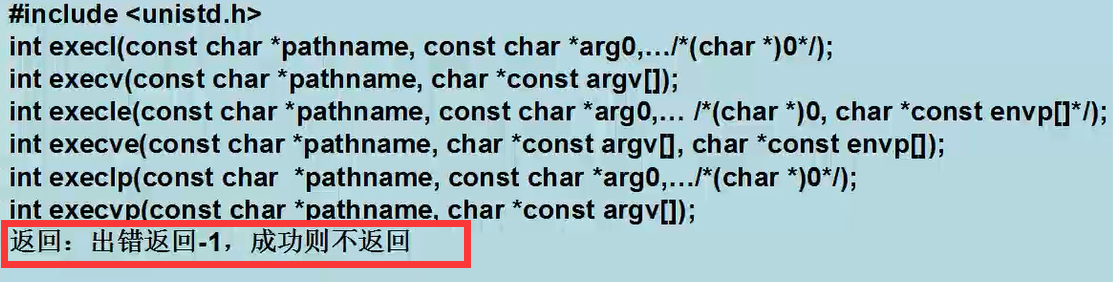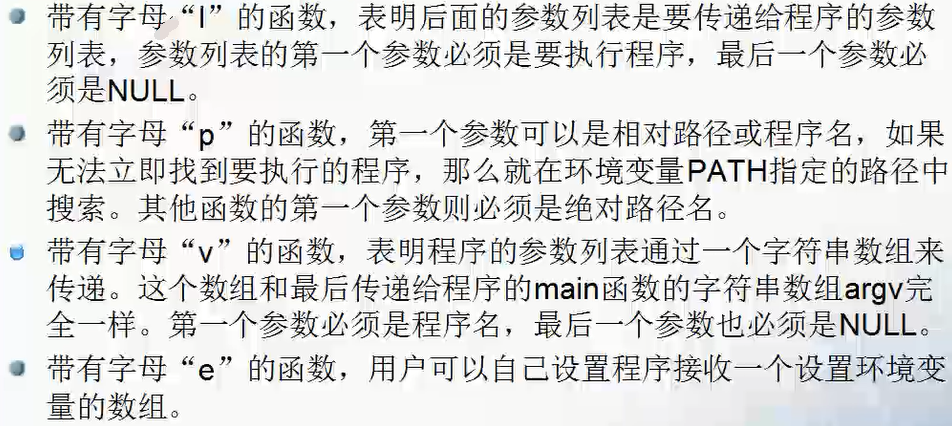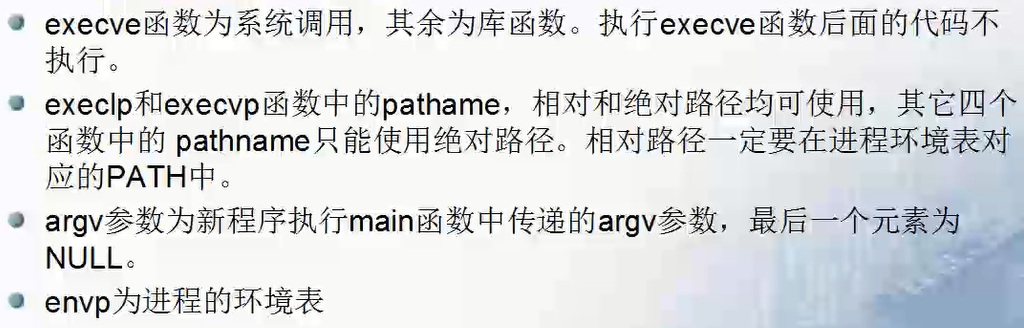exec系列函数关系剖析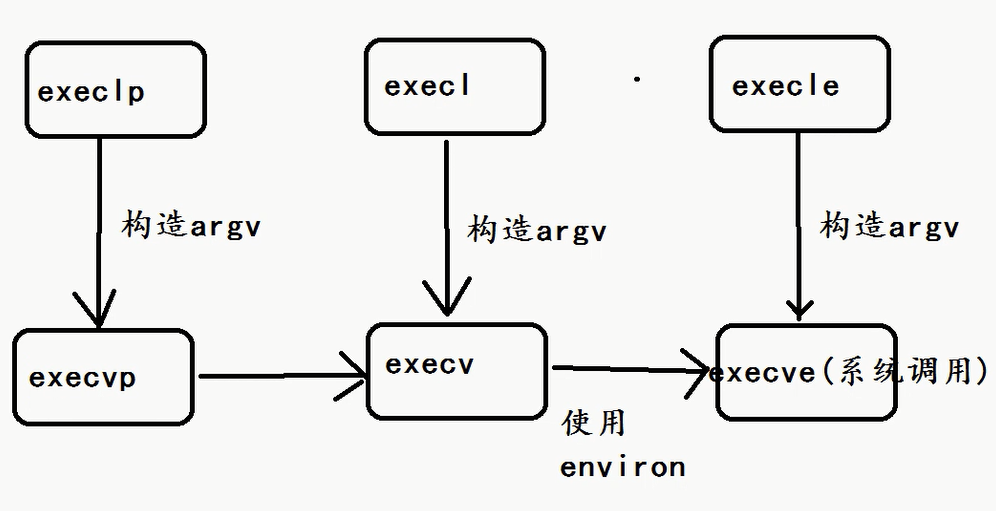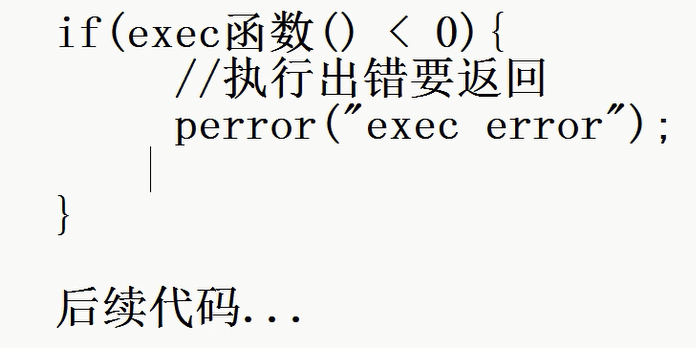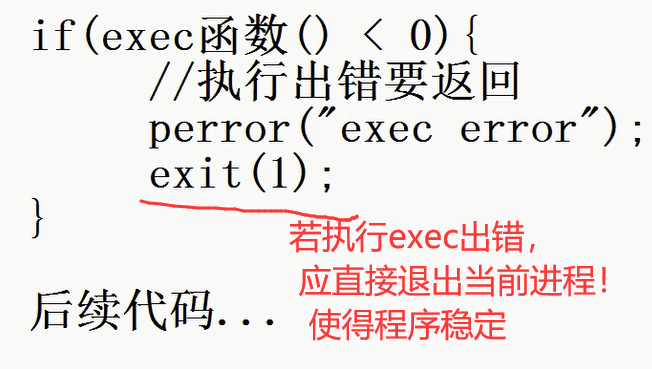PART2

#include <stdio.h>
#include <unistd.h>
#include <stdlib.h>
#include <sys/wait.h>

char* elf_name = "do cat";

char* path1 = "ls";      // 相对路径
char* path2 = "/bin/ls"; // 绝对路径

char* argv1 = "-al";
char* argv2 = "a.out";

int main(void)
{
int status = 0;
pid_t pid;
printf("main-process-pid: %ld\n", (long)getpid());

pid = fork();
if (pid < 0) {
printf("fork error");
}
else if (pid == 0) {

printf("son-process-pid: %ld\n", (long)getpid());
if(execl(path1, elf_name, argv1, argv2, NULL)<0){
perror("execl error");
exit(1);
}else{
printf("execl %s success\n", elf_name);
}
}

wait(NULL);

printf("-------main process ending------\n");

return 0;
}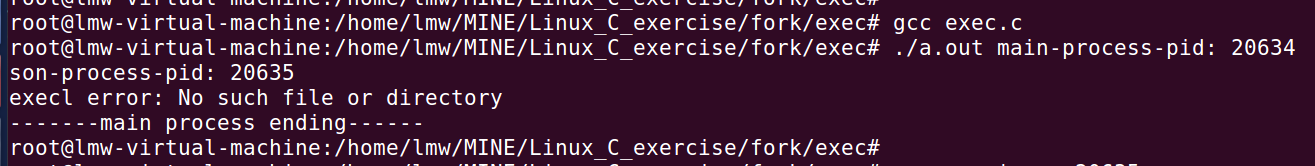#include <stdio.h>
#include <unistd.h>
#include <stdlib.h>
#include <sys/wait.h>

char* elf_name = "do cat";

char* path1 = "ls";      // 相对路径
char* path2 = "/bin/ls"; // 绝对路径

char* argv1 = "-al";
char* argv2 = "a.out";

int main(void)
{
int status = 0;
pid_t pid;
printf("main-process-pid: %ld\n", (long)getpid());

pid = fork();
if (pid < 0) {
printf("fork error");
}
else if (pid == 0) {

printf("son-process-pid: %ld\n", (long)getpid());
if(execl(path2, elf_name, argv1, argv2, NULL)<0){
perror("execl error");
exit(1);
}else{
printf("execl %s success\n", elf_name);
}
}

//wait(NULL); 这里不回收子进程
while(1);

return 0;
}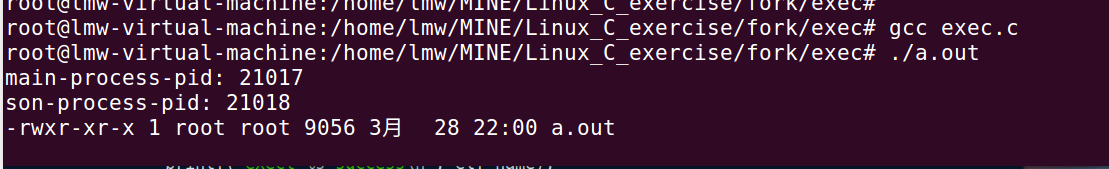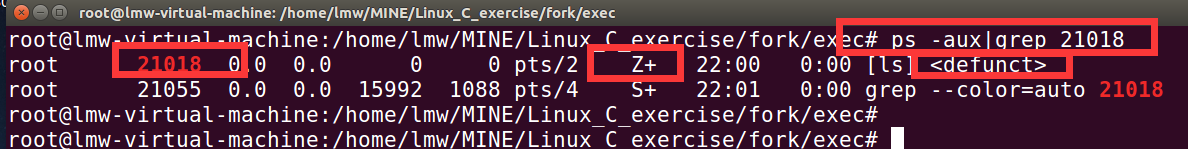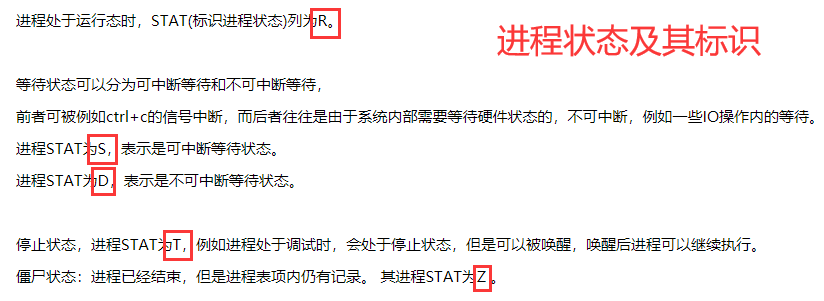本实验和1-1极其相似，故省略。

hello.c :

#include <stdio.h>
#include <unistd.h>

int main(){

sleep(5);

printf("---<hello>elf, is ending---\n");

return 0;
}

exec.c:

#include <stdio.h>
#include <unistd.h>
#include <stdlib.h>
#include <sys/wait.h>

char* elf_name = "do hello";
char* path = "/home/lmw/MINE/Linux_C_exercise/fork/exec/hello"; // 绝对路径

int main(void)
{
int status = 0;
pid_t pid;
printf("main-process-pid: %ld\n", (long)getpid());

pid = fork();
if (pid < 0) {
printf("fork error");
}
else if (pid == 0) {

printf("son-process-pid: %ld\n", (long)getpid());
if(execl(path, elf_name, NULL, NULL, NULL)<0){
perror("execl error");
exit(1);
}else{
printf("execl %s success\n", elf_name);
}
}

wait(NULL);
printf("father waits son successfully \n");

return 0;
}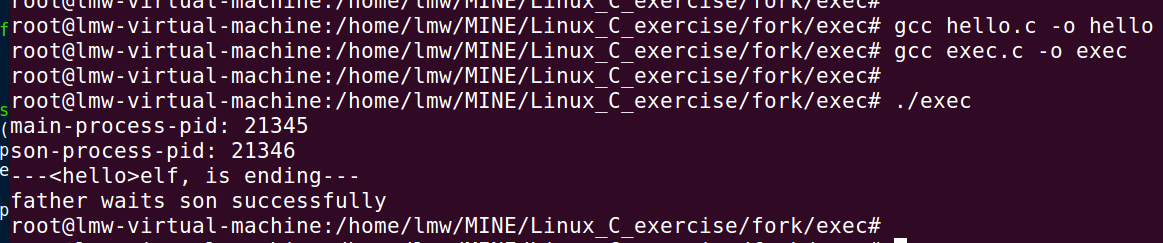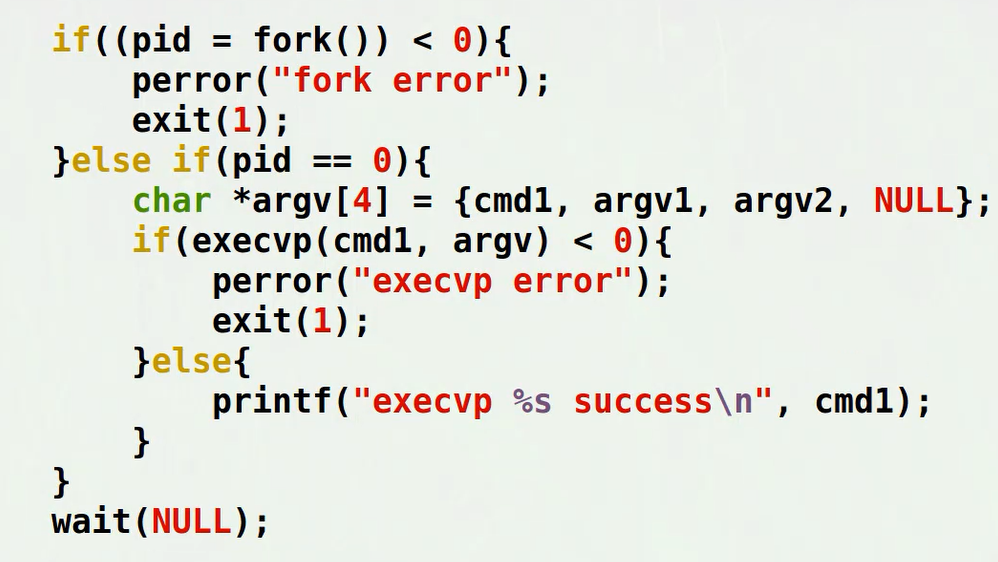Linux下查看系统环境表中的路径：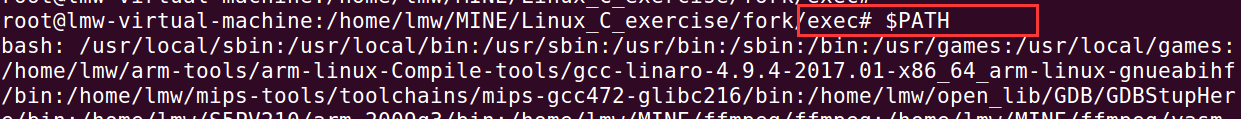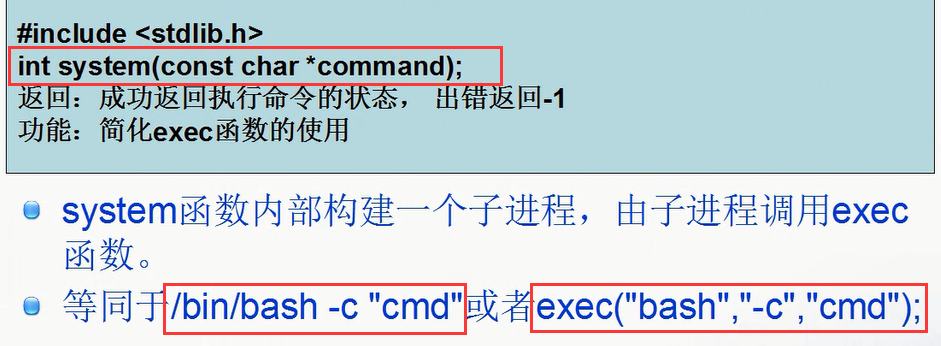#include <stdio.h>
#include <unistd.h>
#include <stdlib.h>
#include <sys/wait.h>

char* test_cmd = "ps";

void mysystem(char* cmd){

pid_t pid;
//printf("main-process-pid: %ld\n", (long)getpid());

pid = fork();
if (pid < 0) {
printf("fork error");
}
else if (pid == 0) {

//printf("son-process-pid: %ld\n", (long)getpid());
if(execlp("bash", "I am bash", "-c", cmd, NULL)<0){
perror("execl error");
exit(1);
}
//printf("mysystem success \n");

}

wait(NULL);
}

int main(void)
{
mysystem(test_cmd);

return 0;
}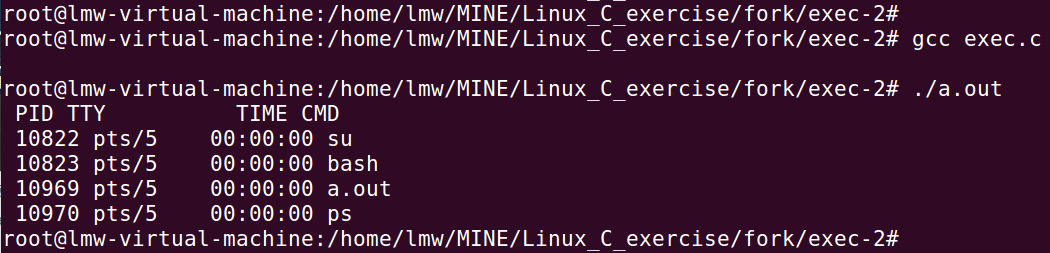.

/************* 社会的有色眼光是：博士生、研究生、本科生、车间工人; 重点大学高材生、普通院校、二流院校、野鸡大学; 年薪百万、五十万、五万; 这些都只是帽子,可以失败千百次，但我和社会都觉得，人只要成功一次，就能换一顶帽子，只是社会看不见你之前的失败的帽子。 当然，换帽子决不是最终目的，走好自己的路就行。 杭州.大话西游 *******/
posted @ 2021-03-29 08:45  一匹夫  阅读(186)  评论(0编辑  收藏  举报